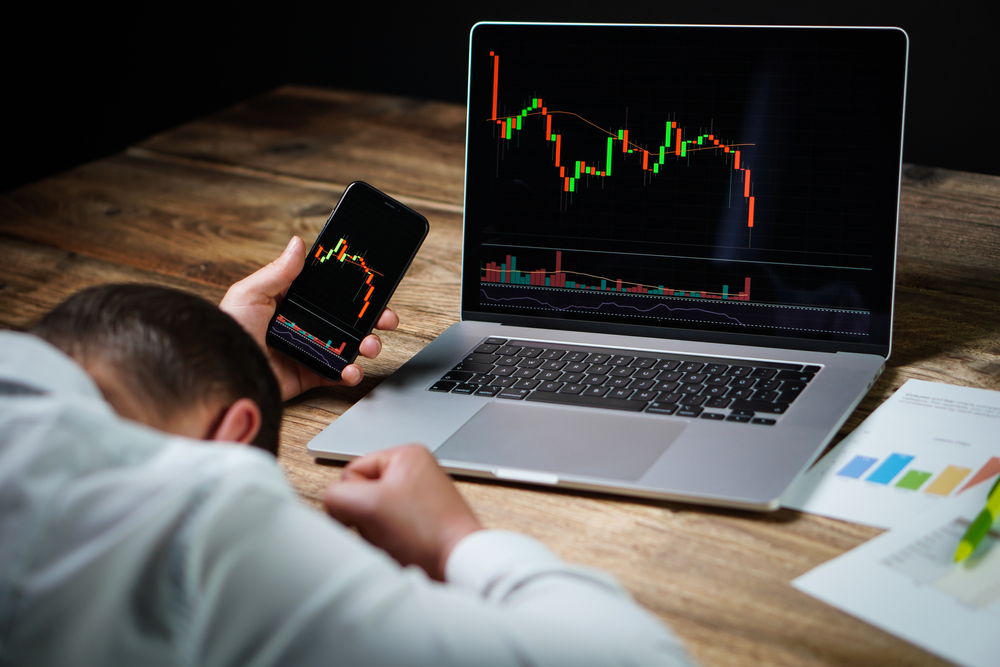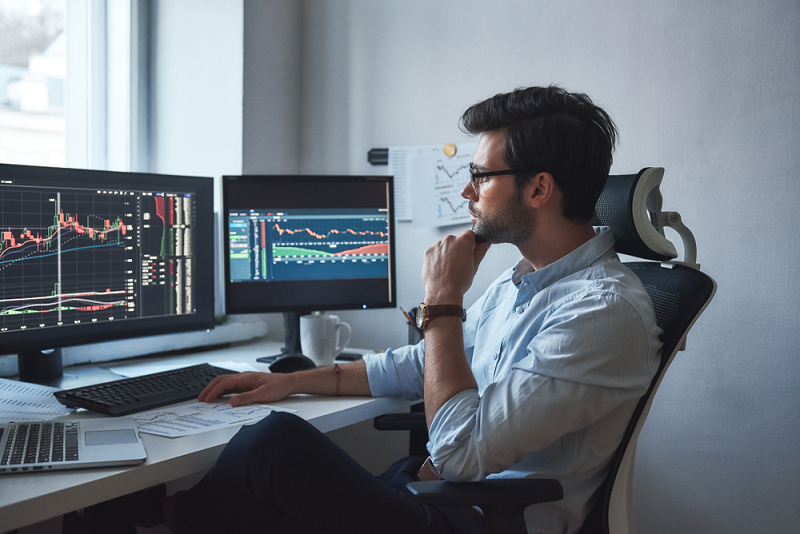Posted by & filed under Trading articles.

The risk of ruin in Forex trading is the statistical measure of the risk that you are willing to take before you stop trading.

For instance, the risk of ruin of 25% on a \$20,000 trading capital tells you to stop trading after losing \$5,000. The risk of ruin is not necessarily the 100% amount of your trading capital but is the maximum amount that you decide to risk and want to stop trading after losing that.

This amount can be substantial to the extent that you may not recover your losses at all. Therefore, understanding the risk of ruin and deciding a percentage is crucial to managing the risk of Forex trading. The risk of ruin is a vast topic and before going into more details, let’s see how it is calculated.

### How to Calculate the Risk of Ruin?

You can calculate the risk of ruin in several ways. In this article, we will use the following formula by Perry J. Kaufman.

((1 – (W – L)) / (1 + (W – L)))^U

To understand the formula, let’s assume that you are trading with \$100,000 and you want to calculate the risk of ruin using a maximum drawdown of 15% or \$15,000.

Now the first thing to calculate is the value of U which requires the risk per trade. Assuming that, you trade with 1% or \$1,000 risk per trade, the value of the U will be 15 (15,000/1,000) consecutive losing trades to reach the maximum drawdown.

The W in the formula represents the percentage of winning trades. The L represents the percentage of losing trades. Let’s say, you have a 60% winning and a 40% loss ratio.

So, the W is 60 or 0.6 while the L is 40 or 0.4. Now if you put all the numbers in the formula you get a number of 5.2% (rounded up to the nearest number). It means there is a 5.2% probability of losing \$15,000 and reaching the risk of ruin.

Now that you understand how the Risk of Ruin is calculated let’s understand its importance in trading.

### The Importance of Risk of Ruin and Risk Management

The risk of ruin can play an important role in your overall risk management strategy. Imagine that you want to reach a destination and you are driving on an unknown road with no directions.

If you are lucky, you may reach your destination but in most cases, you will either be late or not reach your destination at all. Trading Forex without knowing your risk especially the risk of ruin is like driving that unknown road without any direction.

Understanding the risk of ruin will empower you to trade with confidence and help you achieve your trading goals in accomplished manners. You will exactly know where your point of no return is and if you are heading towards that, you will most likely take a break from trading.Traders are prone to risk and managing the risk is the key to success. However, this is easier said than done. At times, you can be right with your prediction but it may turn out to be rocky with short-term losses.

So, you may have to use different methods to cover your losses and stay on the right side of the market. Let’s understand some of the ways you can use to avoid the risk of ruins.

### How to Improve the Risk of Ruins Probability?

The Risk of ruins was initially used in the gambling industry where the payout is usually 1:1. Meaning, if you place a bet, you will win or lose the same amount.

However, in the financial industry, the risk and reward are not always the same and the probability of ruin can increase despite achieving the winning ratio you initially planned. It happens when you don’t achieve your profit targets and close your trades early in small profits.

In other words, you lower the risk to reward ratio. This way even if you achieve the 60% winning ratio the losses from 40% losing trades will exceed your profit. So, it’s important not to unnecessarily close the trades in small profit and maintain your risk to reward ratio.

Another way of improving the odds in your favor is by raising your winning rate. Raising the winning rate by just 5% will greatly lower the probability of ruins.